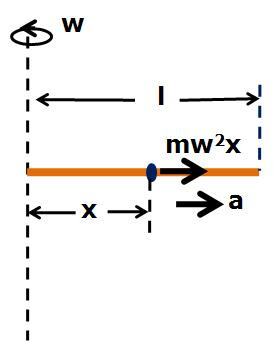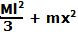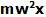## Friday, February 13, 2009

### Irodov Problem 1.272The centrifugal force acting on the sleeve pushes it radially outwards along the rod. Since there are no torques acting on the system about the axis of rotation, the total angular momentum of (sleeve + rod) system must be conserved at all times. As the sleeve moves outwards, the moment of inertia of the (sleeve + rod) system increases. This means that as the sleeve moves out the angular velocity of the spinning rod will decrease. This is similar to a skaters spinning slowly as they extend their arms outwards.

Suppose that at some instant of time the mass m is at a distance x from the the axis of rotation. Further, suppose that at this instant, the angular velocity of the spinning rod is w and that the outward radial acceleration of the sleeve be a. The moment of inertia of the (sleeve + rod) system is given by(see the list). The moment of inertia of the (sleeve + rod) system will be. Initially x=0 and the angular velocity at this time was wo. Since, angular velocity must be conserved, we have,There is only one force acting on the sleeve - the centrifugal force(the sleeve is at a distance x from the axis of rotation) and this results in the radial acceleration a. So we have,Here, v is the radial outward velocity. From (1) and (2) we have,Anonymous said...

sir cant we substitute "w" for "v/l" and "x" for "l" in eq (1)...we get the answer but is it the correct method??..or integration is necessary??

Krishna Kant Chintalapudi said...

No it is not correct.

w = vr is for when the v is the tangential velocity and r is the radius. In this problem v is the radial velocity i.e. velocity of the sleeve along the rod and not tangential velocity.

The use of that formula in this problem the way you suggest is incorrect.Anonymous said...

oh k...got it...thanks..